• Slides: 8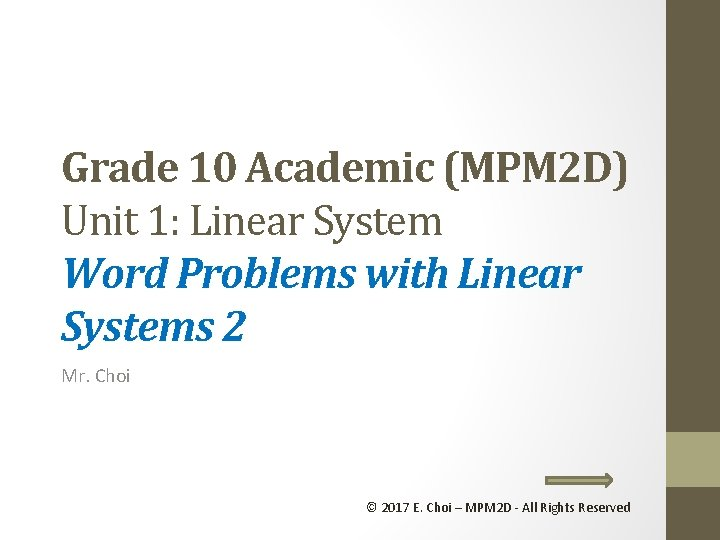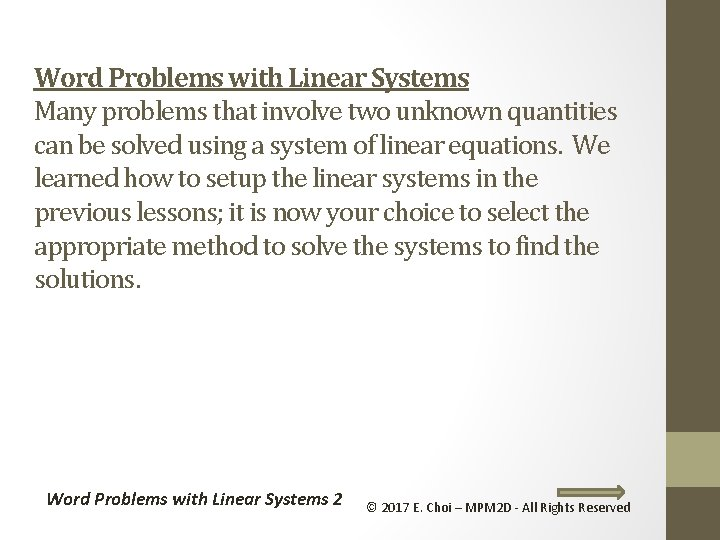Word Problems with Linear Systems Many problems that involve two unknown quantities can be solved using a system of linear equations. We learned how to setup the linear systems in the previous lessons; it is now your choice to select the appropriate method to solve the systems to find the solutions. Word Problems with Linear Systems 2 © 2017 E. Choi – MPM 2 D - All Rights ReservedExample 1: Time, Speed and Distance problems. (Method 1) Sam travelled from Winnipeg to Calgary, a distance of 1210 km, partly by train and partly by bus. The train travels at 90 km/h and the bus travels at 80 km/h. If the total trip takes 14 hours, how far did he travel by train? Distance Let x represent the time spent on the train in hours Let y represent the time spent on the bus in hours Speed 90 80 //////// Train Bus Total Speed Time Distance 14 1210 (2) Sam spend 9 hours on train (810 km) and 5 hours on bus (400 km). Word Problems with Linear Systems 2 © 2017 E. Choi – MPM 2 D - All Rights Reserved TimeExample 1: Time, Speed and Distance problems. (Method 2) Sam travelled from Winnipeg to Calgary, a distance of 1210 km, partly by train and partly by bus. The train travels at 90 km/h and the bus travels at 80 km/h. If the total trip takes 14 hours, how far did he travel by train? Distance Let x represent the distance spent on the train in km Let y represent the distance spent on the bus in km Train Speed 90 Bus 80 Total //////// Speed Distance Time 1210 14 (2) Sam travelled 810 km on train and 400 km on bus. Word Problems with Linear Systems 2 © 2017 E. Choi – MPM 2 D - All Rights Reserved Time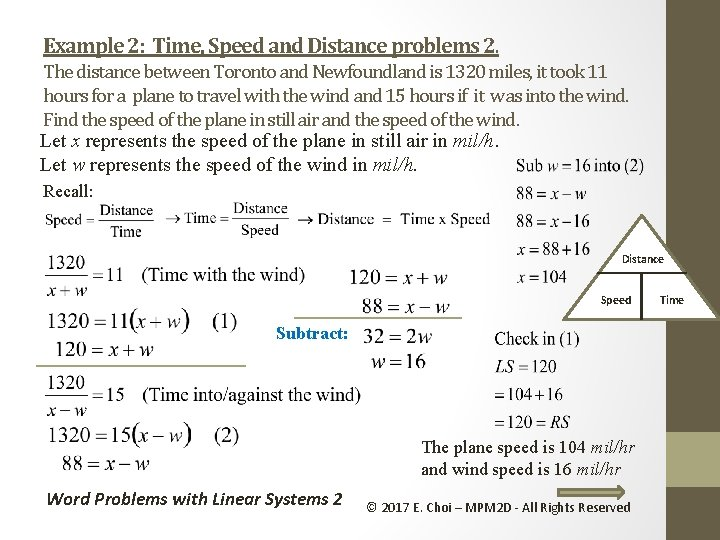Example 2: Time, Speed and Distance problems 2. The distance between Toronto and Newfoundland is 1320 miles, it took 11 hours for a plane to travel with the wind and 15 hours if it was into the wind. Find the speed of the plane in still air and the speed of the wind. Let x represents the speed of the plane in still air in mil/h. Let w represents the speed of the wind in mil/h. Recall: Distance Speed Subtract: The plane speed is 104 mil/hr and wind speed is 16 mil/hr Word Problems with Linear Systems 2 © 2017 E. Choi – MPM 2 D - All Rights Reserved Time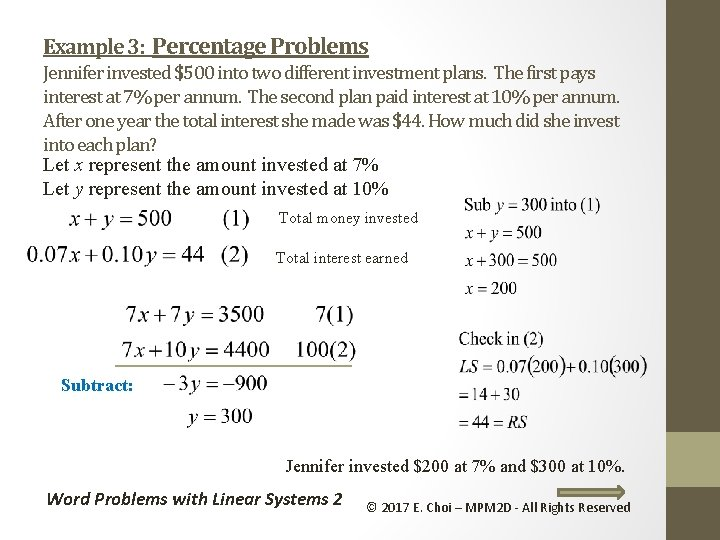Example 3: Percentage Problems Jennifer invested \$500 into two different investment plans. The first pays interest at 7% per annum. The second plan paid interest at 10% per annum. After one year the total interest she made was \$44. How much did she invest into each plan? Let x represent the amount invested at 7% Let y represent the amount invested at 10% Total money invested Total interest earned Subtract: Jennifer invested \$200 at 7% and \$300 at 10%. Word Problems with Linear Systems 2 © 2017 E. Choi – MPM 2 D - All Rights Reserved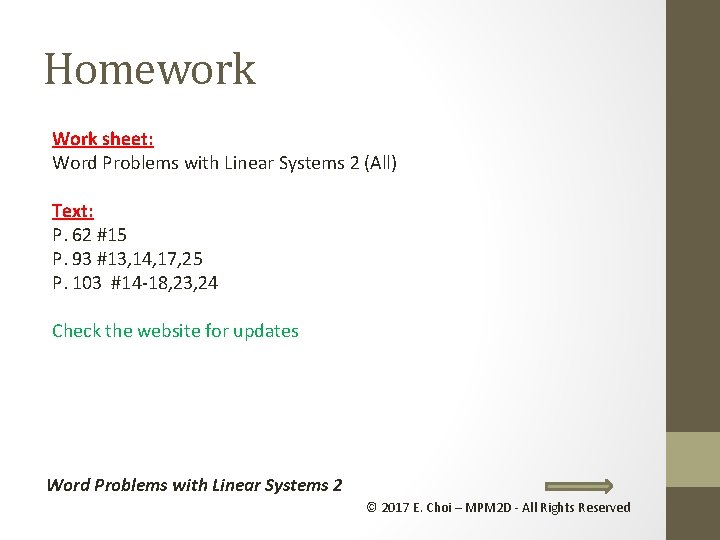Homework Work sheet: Word Problems with Linear Systems 2 (All) Text: P. 62 #15 P. 93 #13, 14, 17, 25 P. 103 #14 -18, 23, 24 Check the website for updates Word Problems with Linear Systems 2 © 2017 E. Choi – MPM 2 D - All Rights Reserved